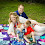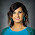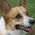## 7.29.2014

### #TMC14 Algebra 2 Session

What can be a connecting thread through Algebra 2?
• Color coding units
• Family of functions
• All equations first, then graphically
• Consistency of vocabulary
• (h, k) forms

Students have to write every function in all three forms: standard, vertex, and intercept. Then identify all key features, intercepts, AoS, asymptotes, end behaviors, vertex, translation from parent function, domain/range, and stretch/compression factor. If it does not exist, write that and explain why.

No canceling! You are either making one or making zero with equations.

For intercepts, be specific. Make students write "Let x = 0, therefore y = __. (x, y)"

Developing rational functions in (h,k) form justifies long division. We use long division to convert rationale into (h,k) form.

(Insert plug for Nix the Tricks) Your past teachers taught you a rule that has now expired.

Trouble with domain and range? Have students use Desmos to write their names with lines.

Display a free virtual TI on your SMART board. Desman is a good intro to Desmos.

It's not the math that's changing, it's the picture. You are applying the same thing over and over.

Use Desmos sliders to show the difference between -2x^3 and (-2x)^3. And to graph all functions and transform at the same time.

Use onlinetonegenerator.com to create tones from trig functions.

Use "rate of change" more than you use "slope". It matters for trig.

Friday- Jonathan Claydon

Break functions into two clusters. First, linear, quadratic, radical, square root (mix in inequalities). Second, log, exponential, rational, polynomial. Start with solving basic types of the first four. Then cycle back and do intermediate types. Cycle back a third time and teach advanced types. Six weeks of equations and four weeks of graphs, the whole semester. End with conics, sequences, and series.

Start January with second cluster. Use graphs to motivate higher algebra. Inverses, transformations, quadratic formula, systems, complex numbers. Complex numbers are what happens when we force the algebra. When two graphs don't intersect, inverting the first graph and finding the intersection gives you the imaginary number solutions. Students use graphs to validate their algebraic answers.

Use data from data.worldbank.org for growth and decay for modeling. Compare countries and growth rates to interpret graphs and motivate extension questions. Use credit card bills and real estate websites for exponentially.

Resources: infinitesums.com/alg2 Open the calendar in Dropbox for pacing guide. DIY guide for goals and practice problems. Independent practice for just problems. All assessments, including finals and finals review are posted. Financial formulas: log gives number of months to pay off, second gives mortgage payment.

Project Ideas: function panels, estimation wall, fact sheets after each concept.

Doesn't do homework, does interactive notebooks, used estimation180 or visual patterns.org for daily warm ups.

Modeling

It's our job to pick problems where variables can be idealized with a mathematical formula.

Take graphing stories and extend it. What can we ask? Volume vs time

What is the area under the curve? (Triangle shape) What is the rate of filling? At that rate, how long would it take to fill the school?

Absement is the absence of displacement, or the filling of the container. A hydraulophone is a real world example.

Try teacher.desmos.com for more class activities

1.ahh! Needed this! Thanks for such good notes :) I'm writing a pacing guide for Glenn's method

1.Aww man...I want to mix Glenn and Jonathan's way together. I like both.

2.I wouldn't mind that, too, just don't know how

3.We should make one together! And teach it together and compare notes ;)

4.You want me to put what I've got in a google doc and we can change and adjust and decide what we think would be best?

5.Heck yes! Are you common core?

6.Yes I am! Is your e-mail misscalculate@gmail.com?

7.Miss.calcul8@gmail

2.These notes are super helpful! I've already gone back to them several times for reference. Thanks so much for taking the time to put these together.

1.Thanks Shelley, glad you can use them. It's the only way I can remember anything! I just type notes in my blog during the session so I can publish later.

3.Thanks for typing these up! It's almost like I was there!